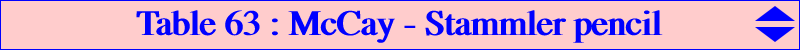The McCay cubic K003 and the decomposed cubic which is the union of the Stammler (diagonal) hyperbola and the line at infinity generate a pencil of stelloids passing through X(3) with tangent the Euler line (except the nodal cubic K258), the in/excenters and the infinite points of K003. It follows that any cubic K(M) of the pencil is entirely characterized by its third point M on the Euler line which is the tangential of X(3) when K(M) is not K258. The radial center X of K(M) is the homothetic of M under h(X3, 1/3). Recall that the polar conic of any point P in K(M) is a rectangular hyperbola and, in particular, that of X(3) is homothetic to the Jerabek hyperbola and its center lies on the line X(3), X(74), X(110), etc. Obviously, K(M) meets this latter polar conic at six points (including X(3)) with tangents concurring at X(3). The contacts lie on a circular nodal quintic with node X(3) (a triple point on the curve), passing through X(1), X(1689), X(1690), X(2574), X(2575), the excenters, the infinite points of K003. The polar conic of M is also a rectangular hyperbola passing through X(3) and homothetic to the Jerabek hyperbola. Similarly, K(M) meets this latter polar conic at six points (including X(3)) with tangents concurring at M. The contacts lie on Q068. Several cubics are already listed in CTC. They are shown in the following table with other remarkable examples. The points X(1), X(3) on K(M) are not repeated.M K(M) X(i) on K(M) for i = X remarks X(4) K003 4, 1075, 1745, 3362 X(2) pK X(20) K077 20, 170, 194, 7991 X(376) X(2) K078 2, 165, 5373, 6194 X(3524) X(3) K100 40, 1670, 1671 X(3) central cubic X(5) K258 5, 39, 2140 X(549) nodal cubic X(546) K910 546, 1385 X(140) X(381) K911 381, 1340, 1341 X(5054) contains the inSteiner foci X(383) 16, 383, 3107 ? X(1080) 15, 1080, 3106 ?